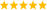# LabVIEW

cancel
Showing results for
Did you mean:

## how to convert acceleration values to speed and position?

We're working on a project that requires acceleration, velocity, and distance data over time.  We're using the NI 9239 in a compact DAQ chassis connected to an acceleromete. There's no problem getting the acceleration data, it's converting it into velocity and distance that's the problem. Do you can help me? thank you very much.Message 1 of 9
(394 Views)

## Re: how to convert acceleration values to speed and position?

Do you know the definitions of acceleration, velocity (not speed), and position?  Have you had a class (or familiarity) with calculus?  Do you understand the concept of integration?  Have you ever learned about digital signal processing and "sampling" (where you approximate a continuous function by measuring it at equally-spaced time intervals)?

If you know at least some of the above, you'll know that velocity is the integral of acceleration, and position is the integral of velocity.  You may also remember from your "Introduction to Calculus" class how you can integrate a function by measuring it at equally spaced intervals and measuring the "area under the curve" (which involves adding the values at the measured points).

One nice thing about LabVIEW is that it is very easy to create tiny little demo programs to generate some data, write code for an algorithm that might compute something for you, and see the result.  Do you know how to use the "Initialize Array" function to create an array with, say, N elements all equal to 9.8 (m/s²) or 32 (ft/s²), the acceleration due to gravity?  Write what you think might be appropriate code to integrate this at 1 second intervals and look at (or, better, plot) what the Velocity would be at 1, 2, 3, ... seconds.  Now integrate once more to get position.  Does this make sense?  Have you ever seen functions like this?  [Have you had high school physics?  Ever heard of Galileo and the Leaning Tower of Pisa?].

Bob Schor

Message 2 of 9
(341 Views)

## Re: how to convert acceleration values to speed and position?

@cong2407 wrote:

There's no problem getting the acceleration data, it's converting it into velocity and distance that's the problem. Do you can help me? thank you very much.

Your picture already shows something that is close. Did you write that? Can you explain what kind of remaining problem you have? (maybe units, etc.?)

As a first step, I would rewrite the VI to simulate some data with known result and get all the math squared away, then hook it back to the accelerometer.

• Are these charts or graphs?
• What is the loop rate?
• How many points do you get per iteration?
• How is the raw data calibrated? Why do you subtract the mean? Is there an arbitrary offset? How does the signal look like before filtering?
2. Explain what you think is wrong
3. Explain what result you get and what you expect to get instead.

It might be clearer to do all math explicit without the use of express VIs, but in the end it should not make a difference.LabVIEW Champion. It all comes together inGCentralWhat does "Engineering Redefined" mean??
Message 3 of 9
(322 Views)

## Re: how to convert acceleration values to speed and position?

I know what you said and I also used the "Initialize Array" function for testing. but the value after the integral is very large.

Message 4 of 9
(280 Views)

## Re: how to convert acceleration values to speed and position?

according to what I have learned, the integral of acceleration is acceleration, the integral of velocity is position. But when I integrate like that, the value after integration increases a lot, leading to the wrong value. can you help me check if i am wrong. below is my VI. thank you!

Message 5 of 9
(274 Views)

## Re: how to convert acceleration values to speed and position?

Hi cong,

@cong2407 wrote:

according to what I have learned, the integral of acceleration is acceleration velocity, the integral of velocity is position. But when I integrate like that, the value after integration increases a lot, leading to the wrong value. can you help me check if i am wrong.

Christian suggested to replace that DAQAssistent by a know signal source, like a simple sine wave:(I removed the loop for simplicity.)

I see two problems:

1. initialization of the filter introduces an unwanted acceleration value.
2. Subtracting a variable mean value in each iteration of your VI will also change the acceleration value in a non-predictable way.

The rest is pure and simple mathematics: a variable offset will kill your integration routines afterwards…

Best regards,
GerdWusing LV2011SP1 + LV2017 (+LV2020 sometimes) on Win10+cRIO
Message 6 of 9
(255 Views)

Message 7 of 9
(241 Views)

## Re: how to convert acceleration values to speed and position?

Hi cong,

@cong2407 wrote:

As has been pointed out in this thread it really helps to get a good understanding of the involved math/signal analysis when you first cleanup that part using a known signal (in the sense of "known expected results") - like a simple sine wave.

When you want to correct your signal analysis using a real-world signal then you need to do that on your own

Best regards,
GerdWusing LV2011SP1 + LV2017 (+LV2020 sometimes) on Win10+cRIO
Message 8 of 9
(222 Views)

## Re: how to convert acceleration values to speed and position?

@cong2407 wrote:

But when I integrate like that, the value after integration increases a lot, leading to the wrong value. can you help me check if i am wrong. below is my VI. thank you!

Does it increase with every iteration? Does it work correctly if you run once without the loop? Your first image shows a displacement on the order of a few cm. What displacement do you expect instead?LabVIEW Champion. It all comes together inGCentralWhat does "Engineering Redefined" mean??
Message 9 of 9
(194 Views)